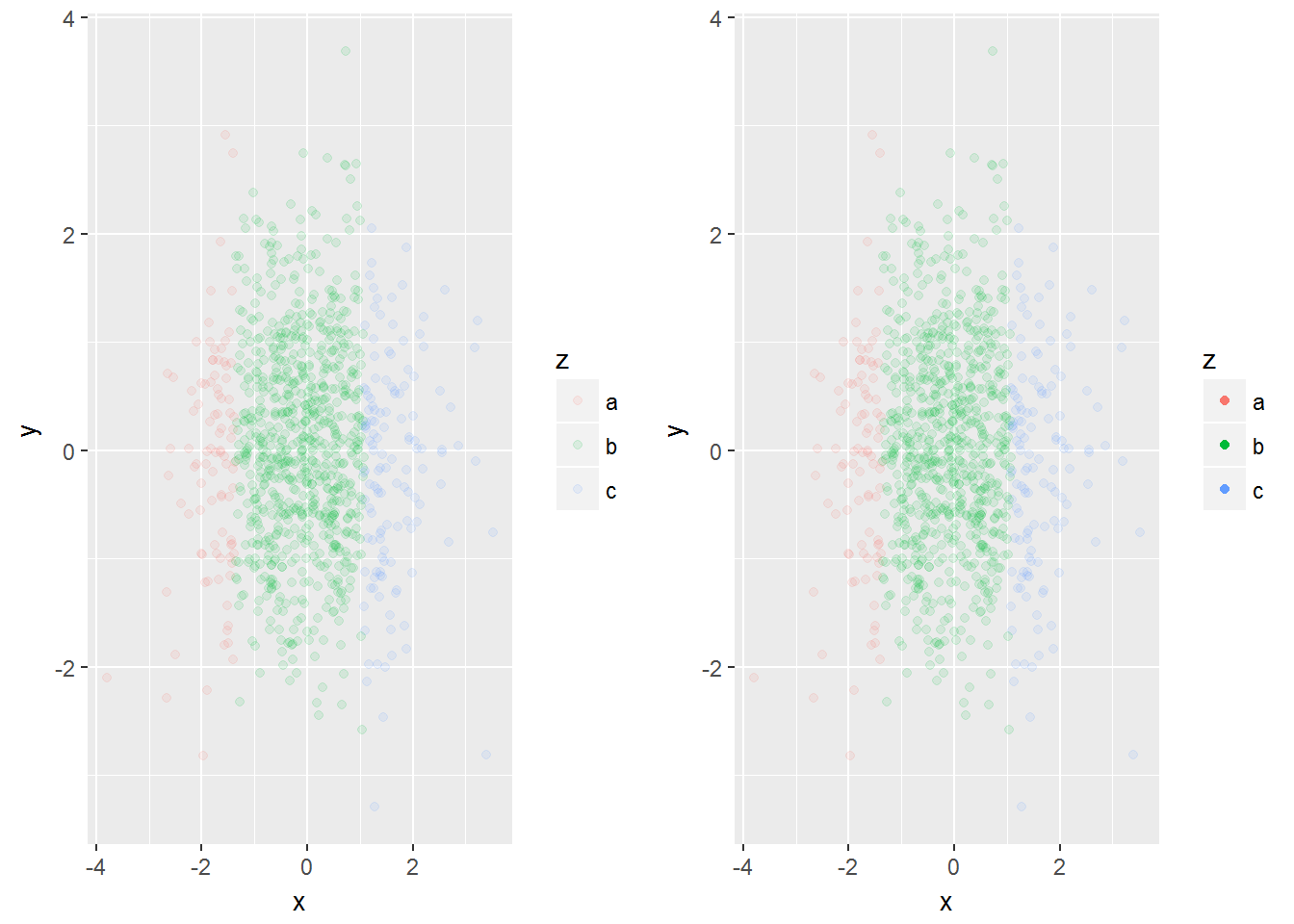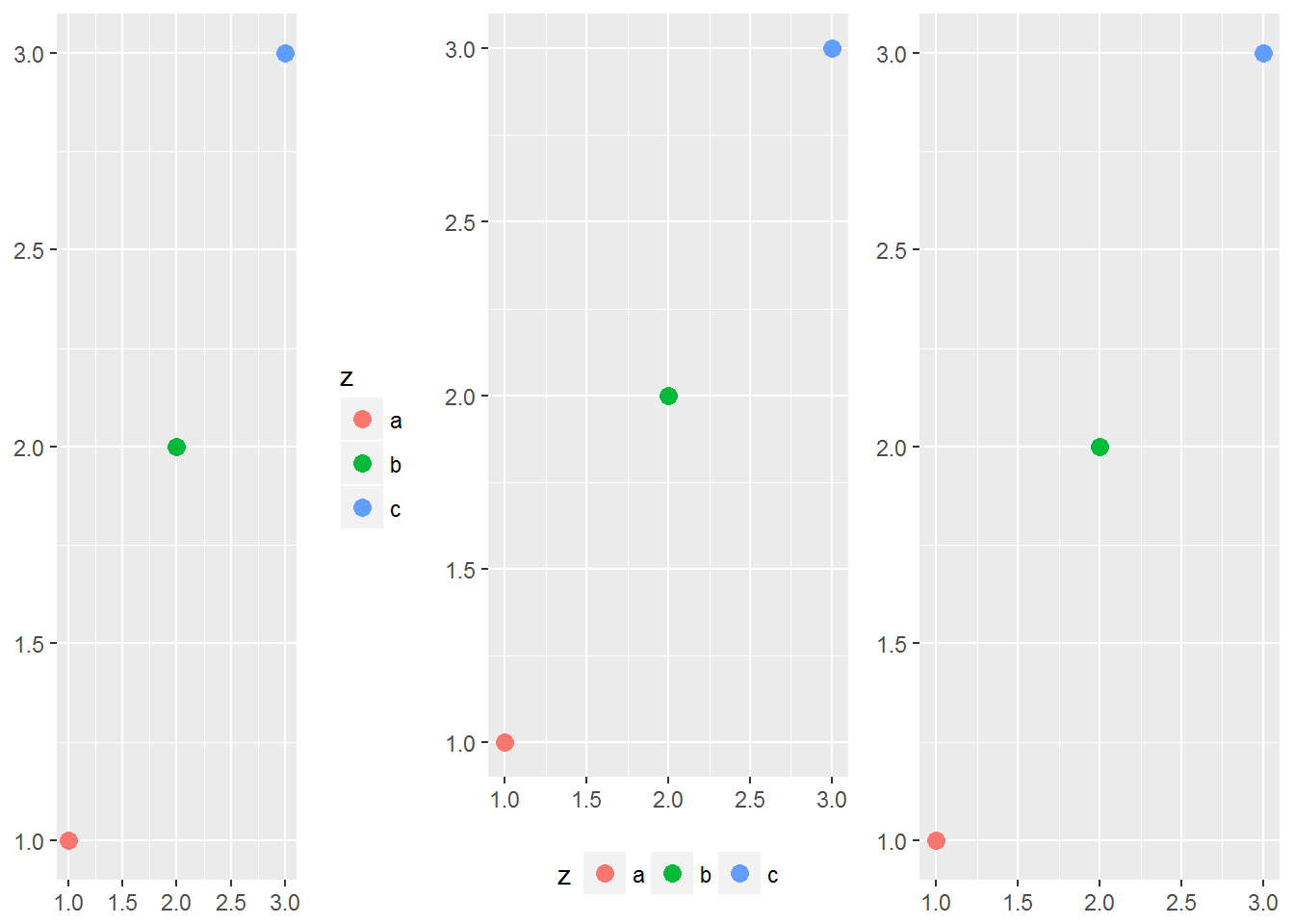# Further Legends

Many of the previous characteristics are the same for both axes and legends. Legends can:

Display multiple aesthetics from multiple layers

Appear in many different locations.

Can be sized and ordered differently.

You can also choose what is placed in a legend. We use the `show.legend` command to do this.

``````ggplot(df, aes(y, y)) +
geom_point(size = 4, colour = "grey20") +
geom_point(aes(colour = z), size = 2)
ggplot(df, aes(y, y)) +
geom_point(size = 4, colour = "grey20",
show.legend = TRUE) +
geom_point(aes(colour = z), size = 2)``````

Sometimes we wish to have the legends display different things. For example if we use transparent colors in the plot we may want solid colors in the legend. The `alpha` command will make the colors more transparent. However we can override the legend colors and set the `alpha` differently:

``````norm <- data.frame(x = rnorm(1000), y = rnorm(1000))
norm\$z <- cut(norm\$x, 3, labels = c("a", "b", "c"))
ggplot(norm, aes(x, y)) +
geom_point(aes(colour = z), alpha = 0.1)
ggplot(norm, aes(x, y)) +
geom_point(aes(colour = z), alpha = 0.1) +
guides(colour = guide_legend(override.aes = list(alpha = 1)))``````

The graph below shows the graph on the left where we let R create the legend. Then the graph on the right shows our changes and overriding on the colors.## Legend Layouts

We can choose the specific layout of the legend. For example we can place it at the “top”, “bottom”, “right” , “left” or not even have a legend at all.

``````df <- data.frame(x = 1:3, y = 1:3, z = c("a", "b", "c"))
base <- ggplot(df, aes(x, y)) +
geom_point(aes(colour = z), size = 3) +
xlab(NULL) +
ylab(NULL)
base + theme(legend.position = "right") # the default
base + theme(legend.position = "bottom")
base + theme(legend.position = "none")``````